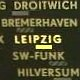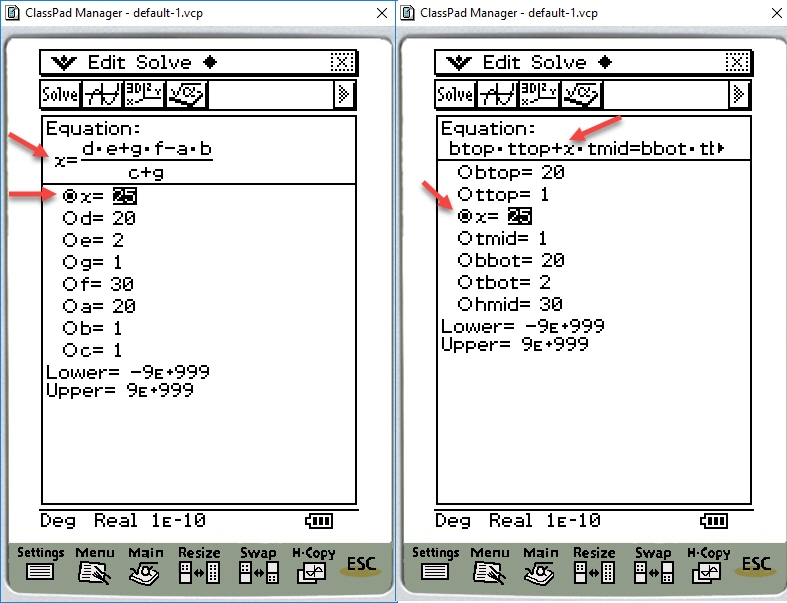# Help for programming to cplua

9 replies to this topic

### #1panda2

panda2

Newbie

•• Members
•• 9 posts
• Gender:Male
• Location:iran,tehran

• Calculators:

Posted 30 January 2018 - 10:53 PM

I will writting a program to cplua,According to this formula

x-4*z=2+x

x=?

Thank you for helping me

### #2piu58

piu58

Casio Freak

•• Members
•• 142 posts
• Gender:Male

• Calculators:
Casio fx-9860G, Casio fx-CG20

Posted 31 January 2018 - 12:16 PM

The formula is only valid for z=-0.5. It is valid for every x then.

### #3panda2

panda2

Newbie

•• Members
•• 9 posts
• Gender:Male
• Location:iran,tehran

• Calculators:

Posted 31 January 2018 - 11:59 PM

The formula is only valid for z=-0.5. It is valid for every x then.

Hi،Thanks, yes, I made a mistake,please guide me for writting this formula,

x=tonumber(input("x?"))
y=tonumber(input("y?"))
x-4*z=2*x+y

What do I need to write to get z ?

### #4piu58

piu58

Casio Freak

•• Members
•• 142 posts
• Gender:Male

• Calculators:
Casio fx-9860G, Casio fx-CG20

Posted 01 February 2018 - 05:25 AM

This formula is equivalent to z=-0.25(x+y)

You don't need a program for that.

• panda2 likes this

### #5panda2

panda2

Newbie

•• Members
•• 9 posts
• Gender:Male
• Location:iran,tehran

• Calculators:

Posted 01 February 2018 - 04:02 PM

This formula is equivalent to z=-0.25(x+y)

You don't need a program for that.

Tnx,However, when the formula is larger, it is no longer possible to bring the unknown one first

### #6piu58

piu58

Casio Freak

•• Members
•• 142 posts
• Gender:Male

• Calculators:
Casio fx-9860G, Casio fx-CG20

Posted 01 February 2018 - 08:19 PM

You always may try to resolve the formula partially to the variable looked for. And the use a starting value and calculate the formula again and again until it converges. If it diverges, resolve to another value of the variable looked for.

• panda2 likes this

### #7panda2

panda2

Newbie

•• Members
•• 9 posts
• Gender:Male
• Location:iran,tehran

• Calculators:

Posted 02 February 2018 - 12:20 AM

You always may try to resolve the formula partially to the variable looked for. And the use a starting value and calculate the formula again and again until it converges. If it diverges, resolve to another value of the variable looked for.

Thank you, but this is difficult, for example in the formula if x is unknown, whether simple formula that breaks down,Then this method will not be easy; ; I want to answer every variable in the formula.

for exp: (btop×ttop)+(x×tmid)=(bbot×tbot)+((hmid-x)×tmid)

x=?

How to write this formula in the program of cplua or basic

Edited by panda2, 02 February 2018 - 12:22 AM.

### #8piu58

piu58

Casio Freak

•• Members
•• 142 posts
• Gender:Male

• Calculators:
Casio fx-9860G, Casio fx-CG20

Posted 02 February 2018 - 05:42 AM

Again simple. You dnon't need a claculator program but a deeper understanding of mathematics.

For clarity I replaced

btop a

ttop b

tmid c

bbot d

tbot e

hmid f

tmig g

ab+xc = de+gf-gx

and resolves to

x = (de+gf-ab) / (c+g)

(if I have done all correctly).

• panda2 likes this

### #9panda2

panda2

Newbie

•• Members
•• 9 posts
• Gender:Male
• Location:iran,tehran

• Calculators:

Posted 02 February 2018 - 05:12 PM

Again simple. You dnon't need a claculator program but a deeper understanding of mathematics.

For clarity I replaced

btop a

ttop b

tmid c

bbot d

tbot e

hmid f

tmig g

ab+xc = de+gf-gx

and resolves to

x = (de+gf-ab) / (c+g)

(if I have done all correctly).

Thank you, your method is correct, but anyway, there must be a way to get the unknown as a solve of classpad, instead of simplifying the formula.If the formula is long, this simplifies the problemEdited by panda2, 02 February 2018 - 05:28 PM.

### #10panda2

panda2

Newbie

•• Members
•• 9 posts
• Gender:Male
• Location:iran,tehran

• Calculators:

Posted 02 February 2018 - 07:37 PM

I found the solution

ClrText
print"hello"
input x
input z
Print solve(z=x+y,y)

#### 0 user(s) are reading this topic

0 members, 0 guests, 0 anonymous users